Skip to main content

# Magnetic geodesics on surfaces with singularities

## Abstract

We focus on the numerical study of magnetic geodesics on surfaces, including surfaces with singularities. In addition to the numerical investigation, we give restrictive necessary conditions for tangency directions of magnetic geodesics passing through certain types of singularities.

## Introduction

A magnetic geodesic describes the trajectory of a charged particle in a Riemannian manifold M under the influence of an external magnetic field. Numerical experimentation suggests that almost all magnetic geodesics tend to avoid any lightlike singularities (points where the tangent spaces are lightlike) that M may have, regardless of choice of bounded smooth external magnetic field. Our primary result is a mathematically rigorous confirmation of this behavior.

Initially, we take M to be a complete, orientable Riemannian manifold without boundary of dimension n and Riemannian metric 〈·,·〉. For a given two-form Ω defined on M we associate a smooth section ZHom(T M,T M) defined via

$$\langle\eta,Z(\xi)\rangle=\Omega(\eta,\xi)$$

for all η,ξTM. We will investigate the existence of closed curves γ=γ(t) satisfying the following equation

$$\nabla_{\gamma^{\prime}} \gamma^{\prime} = Z\left(\gamma^{\prime}\right).$$
(1.1)

Note that, in contrast to geodesics, which correspond to Z=0, the equation for magnetic geodesics is not invariant under rescaling of t.

In the case that M is a surface, that is n=2, we know that every two-form Ω is a multiple of the volume form Ω 0 associated with 〈·,·〉. Thus, every two-form can be written as Ω=κ Ω 0 for some function $$\kappa \colon M\to \mathbb {R}$$. We can exploit this fact to rewrite the right hand side of (1.1) as

$$Z\left(\gamma'\right)=\kappa J^{90}_{\gamma}\left(\gamma'\right),$$
(1.2)

where $$J^{90}_{\gamma }$$ represents rotation in the tangent space T γ M by angle π/2, see . Due to this fact one often refers to (1.2) as the prescribed geodesic curvature equation, and κ is proportional to the geodesic curvature function.

We will always assume that κ is a smooth and bounded function.

### Remarks 1.1

If the two-form Ω is exact, then (1.1) also arises from a variational principle, see [ 2 , 14 ].

Note that a solution of (1.1) has constant speed, which follows from

$$\frac{\partial}{\partial t}\frac{1}{2}|\gamma'|^{2}=\left\langle\nabla_{\gamma^{\prime}}\gamma^{\prime},\gamma^{\prime}\right\rangle =\left\langle Z(\gamma'),\gamma^{\prime}\right\rangle=\Omega\left(\gamma^{\prime},\gamma^{\prime}\right)=0$$
(1.3)

due to the skew-symmetry of the two-form Ω.

For magnetic geodesics on surfaces, several existence results are available, employing techniques from symplectic geometry [5,6] and from the calculus of variations . In the papers of Schneider [11,12], and the paper by Schneider and Rosenberg , existence results for closed magnetic geodesics on Riemann surfaces are given by studying the zeros of a certain vector field. Recently, an existence result for magnetic geodesics has been established by the heat flow method .

Here rather, we give an approach more aimed at usefulness for numerics, and then proceed to produce examples of closed magnetic geodesics numerically. We then study the behavior of magnetic geodesics near singular points of a surface by proving our main result Theorem 3.1, and our proof employs the fact that magnetic geodesics have constant speed parametrization.

This article is organized as follows: In Section 2 we derive several numerical examples of magnetic geodesics. Moreover, we provide several analytic statements that support our numerical calculations. In Section 3 we focus on magnetic geodesics on almost-everywhere-spacelike surfaces with lightlike singularities and show that they will tend to turn away from the singularities unless they enter the singular sets at specific angles, which is the content of Theorem 3.1.

## Closed magnetic geodesics on surfaces in Euclidean and Minkowski 3-spaces

Before we turn to the numerical integration of (1.1) let us make the following observations.

By the Theorem of Picard-Lindeloef we always get a local solution to (1.1). However, similar to the classical Hopf-Rinow theorem in Riemannian geometry we can show

### Theorem 2.1

Let (M,〈·,·〉)be a complete Riemannian surface and $$\kappa : M \to \mathbb {R}$$ be a prescribed function. Let

$$\gamma(t) : (a,b) \to M$$

be a curve in M with geodesic curvature κ(γ(t)) at γ(t), in other words, γ is a nontrivial solution to

$$\nabla_{\gamma^{\prime}} \gamma^{\prime} = \kappa J^{90}_{\gamma}\left(\gamma'\right) \;.$$
(2.1)

Then the domain (a,b) can be extended to all of $$\mathbb {R}$$.

### Proof

To show that the maximal interval of existence of (2.1) is indeed all of $$\mathbb {R}$$ we assume that there is a maximal interval of existence and then show that we can extend the solution beyond that interval. Thus, assume that γ:(a,b)→M is a magnetic geodesic with maximal domain of definition. Since |γ |2 is constant we know that the curve γ has constant length L[γ]. Then we have for a sequence $$\gamma (t_{i})_{i\in \mathbb {N}}$$

$$d(\gamma(t_{i}),\gamma(t_{j}))\leq L[\gamma_{[t_{i},t_{j}]}]\leq C|t_{i}-t_{j}|,$$

where d denotes the Riemannian distance function. Hence, $$\gamma (t_{i})_{i\in \mathbb {N}}$$ is a Cauchy sequence with respect to d. It is easy to see that the limit is independent of the chosen sequence.

As a next step, we show that we may extend γ to (a,b]. To this end we use the local expression for (2.1), that is

$${} \left(\gamma^{\prime\prime}\right)^{k}=-\sum_{i,j=1}^{2}\Gamma^{k}_{ij}\left(\gamma^{\prime}\right)^{i}\left(\gamma^{\prime}\right)^{j}-\kappa \left(J^{90}_{\gamma}\left(\gamma'\right)\right)^{k},\qquad k=1,2.$$

Now, consider the expression

$$|\gamma^{\prime}(t_{i})-\gamma^{\prime}(t_{j})|_{L^{\infty}}= \left|\int_{t_{i}}^{t_{j}}\gamma^{\prime\prime}(\tau)d\tau\right|_{L^{\infty}} \leq C|t_{i}-t_{j}|_{L^{\infty}}\,.$$

Using that |γ | is constant it follows that γ (t i ) forms a Cauchy sequence and converges to some $$\gamma ^{\prime }_{\infty }$$. Again, the limit is independent of the chosen sequence.

By differentiating the equation for magnetic geodesics and using the same method as for estimating $$|\gamma ^{\prime }(t_{i})-\gamma ^{\prime }(t_{j})|_{L^{\infty }}$$ we can show that also γ ′′(t i ) forms a Cauchy sequence.

Now, assume that $$\tilde {\gamma }\colon (\beta -a,\beta +a)\to M$$ is a magnetic geodesic with $$\tilde {\gamma }(\beta)=\hat {\gamma }(\beta)$$ and $$\tilde {\gamma }^{\prime }(\beta)=\hat {\gamma }^{\prime }(\beta)$$. Since magnetic geodesics are uniquely determined by their initial values, $$\tilde {\gamma }$$ and $$\hat {\gamma }$$ coincide on their common domain of definition. This yields a continuation of γ as a magnetic geodesic on (a,b+β), which contradicts the maximality of b. □

### Remarks 2.2

We will be looking for closed solutions of (1.1), which Theorem 2.1 does not inform us about. Theorem 2.1 can be generalized to higher dimensions. Note that Theorem 2.1 no longer holds on a surface that is in some way not complete, for example a surface with singularities.

Again, since magnetic geodesics are uniquely determined by their initial values, the intermediate value theorem gives us the following method for finding closed magnetic geodesics, which was employed to produce the numerical examples of closed magnetic geodesics found in the figures in this paper:

### Proposition 2.3

Let n=2. Suppose there exists a continuous one-parameter family of solutions γ s , with s [ 0,1], as in Theorem 2.1, and suppose there exist t 1(s) and t 2(s) in $$\mathbb {R}$$ with t 2(s)>t 1(s) such that

1. (1)

t 1(s) and t 2(s) depend continuously on s,

2. (2)

γ s (t 1(s))=γ s (t 2(s)) for all s [ 0,1],

3. (3)

$$\{ \gamma _{0}^{\prime }(t_{1}(0)), \gamma _{0}^{\prime }(t_{2}(0)) \}$$ spans $$T_{\gamma _{0}(t_{1}(0))}M=T_{\gamma _{0}(t_{2}(0))}M$$ with one orientation, and $$\{ \gamma _{1}^{\prime }(t_{1}(1)), \gamma _{1}^{\prime }(t_{2}(1)) \}$$ spans $$T_{\gamma _{1}\!(t_{1}(1))}M\,=\,T_{\gamma _{1}\!(t_{2}(1))}M$$ with the opposite orientation.

Then γ:[ t 1(s),t 2(s)]→M forms a closed loop for some s(0,1).

For our numerical studies of (1.2) we need the following

### Proposition 2.4

Let $$M\subset \mathbb {R}^{3}$$ be a surface. Then Eq. 1.2 is equivalent to the system

$$\begin{array}{*{20}l} |\gamma'|^{2}&=c~~\text{is constant,} \end{array}$$
(2.2)
$$\begin{array}{*{20}l} \frac{1}{|n|}\left\langle\gamma^{\prime\prime},\gamma'\times n\right\rangle&=\kappa |\gamma'|^{2}, \end{array}$$
(2.3)

where n denotes a normal to the surface compatible with J 90 and × denotes the cross product in $$\mathbb {R}^{3}$$.

### Proof

The first equation can easily be derived from (1.2) (see also Eq. (1.3)):

$$\frac{\partial}{\partial t}\frac{1}{2}|\gamma'|^{2}=\left\langle\nabla_{\gamma'}\gamma',\gamma'\right\rangle =\kappa\left\langle J^{90}_{\gamma}(\gamma'),\gamma'\right\rangle=0.$$

For the second equation, we consider

$${} \frac{1}{|n|}\!\left\langle\gamma^{\prime\prime},\gamma'\times n\right\rangle \,=\, \left\langle\gamma^{\prime\prime}, J^{90}_{\gamma}(\gamma')\! \right\rangle \,=\, \frac{1}{\kappa} \left\langle\gamma^{\prime\prime}, \nabla_{\gamma'}\gamma' \right\rangle \!= \frac{1}{\kappa}\! \left|\nabla_{\gamma'}\gamma'\right|^{2}.$$

Since the magnetic geodesic equation implies $$\phantom {\dot {i}\!}|\nabla _{\gamma '}\gamma '|^{2}=\kappa ^{2} |\gamma '|^{2}$$, we obtain the second equation.

To establish the equivalence between (1.1) and the system (2.2), (2.3) we note that (2.2), (2.3) is obtained from (1.1) by taking the scalar product with both γ and $$J_{\gamma }^{90}(\gamma ')$$. However, $$\gamma ', J_{\gamma }^{90}(\gamma ')$$ form a basis of the tangent space T γ M, yielding the equivalence. □

We now consider a surface S(u,v) parametrized by coordinates (u,v) in a subdomain of $$\mathbb {R}^{2}$$, and a curve γ(t)=S(u(t),v(t)) on the surface. We can rewrite (2.2) and (2.3): Expanding to obtain

\begin{aligned} \gamma'&=S_{u}u'+S_{v}v',\\ \gamma^{\prime\prime}&=S_{uu}u^{\prime2}+S_{vv}v^{\prime2}+S_{u}u^{\prime\prime}+S_{v}v^{\prime\prime}+2S_{uv}u'v' \end{aligned}

and taking n=S u ×S v , and using

$$\gamma'\times n=\gamma'\times (S_{u}\times S_{v}) = \left\langle\gamma',S_{v}\right\rangle S_{u}-\left\langle\gamma',S_{u}\right\rangle S_{v} \;,$$

we can convert Eqs. (2.2) and (2.3) into

{\kern25pt} \begin{aligned} c=|S_{u}|^{2}u^{\prime2}+|S_{v}|^{2}v^{\prime2}+2\langle S_{u},S_{v}\rangle u'v', \end{aligned}
(2.4)
{} \begin{aligned} c |S_{u} \times S_{v}| \kappa=\left(u^{\prime\prime}v'-v^{\prime\prime}u'\right)\left(|S_{v}|^{2}|S_{u}|^{2}-|\langle S_{u},S_{v}\rangle|^{2}\right) \end{aligned}
(2.5)
{\kern35pt} \begin{aligned} &+u^{\prime3}\left(\langle S_{u},S_{v}\rangle\langle S_{uu},S_{u}\rangle-|S_{u}|^{2}\langle S_{v},S_{uu}\rangle\right)\\ &+v^{\prime3}\left(|S_{v}|^{2}\langle S_{vv},S_{u}\rangle -\langle S_{v},S_{u}\rangle\langle S_{v},S_{vv}\rangle\right) \\ &+u^{\prime2}v'\left(|S_{v}|^{2}\langle S_{uu},S_{u}\rangle-\langle S_{u},S_{v}\rangle\langle S_{uu},S_{v}\rangle\right. \\ &\left.+2\langle S_{u},S_{v}\rangle\langle S_{uv},S_{u}\rangle-2|S_{u}|^{2}\langle S_{uv},S_{v}\rangle\right) \\ &+v^{\prime2}u'\left(\langle S_{u},S_{v}\rangle\langle S_{u},S_{vv}\rangle-|S_{u}|^{2}\langle S_{vv},S_{v}\rangle\right.\\ &\left.+2\langle S_{u},S_{uv}\rangle |S_{v}|^{2}-2\langle S_{u},S_{v}\rangle\langle S_{uv},S_{v}\rangle\rangle\right). \end{aligned}

However, if the surface is conformally parametrized, that is

$$\langle S_{u},S_{v}\rangle=0,\qquad |S_{u}|^{2}=|S_{v}|^{2}=f(u,v) \geq 0,$$

the system (2.4) and (2.5) simplifies to

$$\begin{array}{*{20}l} c=&\left(u^{\prime2}+v^{\prime2}\right)f, \end{array}$$
(2.6)
$$\begin{array}{*{20}l} c \kappa=&\left(u^{\prime\prime}v'-v^{\prime\prime}u'\right)f-u^{\prime3}\langle S_{v},S_{uu}\rangle+v^{\prime3}\langle S_{u},S_{vv}\rangle \\ &-\frac{1}{2}u^{\prime2}v'f_{u}+\frac{1}{2}v^{\prime2}u'f_{v}. \end{array}$$
(2.7)

Using the formulations (2.4), (2.5), (2.6) and (2.7), we now use the idea in Proposition 2.3 to numerically produce examples of closed magnetic geodesics.

### 2.1 Example: round sphere

Parameterizing the sphere as

$$S(u,v) = (\cos u \cos v, \cos u \sin v, \sin u) \;,$$

the magnetic geodesic system becomes

{} \begin{aligned} c=&u^{\prime2}+v^{\prime2}\cos^{2}u \;, \\ c \kappa=&\left(u^{\prime\prime}v'-v^{\prime\prime}u'\right)\cos u+v^{\prime3}\cos^{2}u\sin u+2u^{\prime2}v'\sin u \;. \end{aligned}

Note that κ=0 will give great circles of course, and clearly κ a nonzero constant will give a circle in the sphere that is not a great circle. κ= sinu can give a curve as in Fig. 1.Fig. 1

### 2.2 Example: Clifford torus

Parameterizing the Clifford torus by

$${} S(u,v) = \left(\left(\sqrt{2} + \cos u\right) \cos v, \left(\sqrt{2} + \cos u\right) \sin v, \sin u\right)$$

yields the system

{} \begin{aligned} c=&u^{\prime2}+v^{\prime2}\left(\sqrt{2}+\cos u\right)^{2}, \\ c \kappa=&\left(u^{\prime\prime}v'-v^{\prime\prime}u'\right)\left(\sqrt{2}+\cos u\right)\\ &+v'\sin u\left(v^{\prime2}(\sqrt{2}+\cos u)^{2}+2u^{\prime2}\right). \end{aligned}

Two examples of closed geodesics, that is κ=0, are given in Fig. 2. Other examples of closed magnetic geodesics on the Clifford torus are shown in Fig. 3.Fig. 2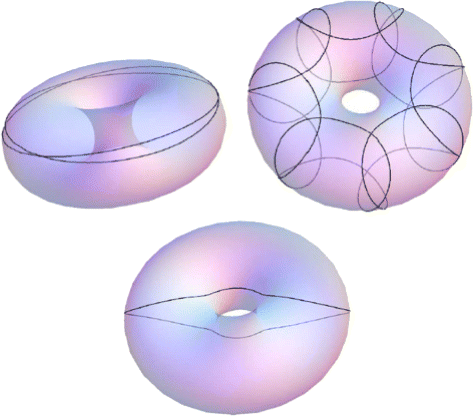Fig. 3

### 2.3 Example: catenoid

Conformally parameterizing the catenoid, with f= cosh2u, as

$$S(u,v)=(\cosh u \cos v, \cosh u \sin v, u) \;,$$

the system becomes

\begin{aligned} &{}c=\left(u^{\prime2}+v^{\prime2}\right)\cosh^{2}u, \\ &{}c \kappa=\left(u^{\prime\prime}v'-v^{\prime\prime}u'\right)\cosh^{2}u - \sinh u\cosh u\left(v^{\prime3}+u^{\prime2}v'\right). \end{aligned}

Examples are found in Fig. 4.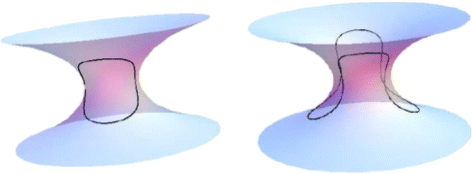Fig. 4

### 2.4 Example: minimal Enneper surface

The Enneper minimal surface in $$\mathbb {R}^{3}$$ can be conformally parametrized as

$${} S(u,v)=\left(u-\frac{1}{3}u^{3}+u v^{2},-v+\frac{1}{3}v^{3}-v u^{2}, u^{2}-v^{2}\right) \;,$$

with f=(1+u 2+v 2)2. This yields the system

{} \begin{aligned} c &=\left(u^{\prime2}+v^{\prime2}\right)\left(1+u^{2}+v^{2}\right)^{2}, \\ \frac{c \kappa}{1+u^{2}+v^{2}}&=\left(u^{\prime\prime}v'-v^{\prime\prime}u'\right)\left(1+u^{2}+v^{2}\right)+2\left(u^{\prime2}+v^{\prime2}\right)\\ &\quad\left(vu'-uv'\right). \end{aligned}

An example is found in Fig. 5.Fig. 5

### 2.5 Minkowski 3-space

Let $$\mathbb {R}^{2,1}$$ denote the Minkowski 3-space $$\{ (x,y,s) \, | \, x,y,s \in \mathbb {R} \}$$ with Lorentzian metric of signature (+,+,−). Spacelike surfaces with mean curvature identically zero are called maximal surfaces, and the next example is such a surface. Our primary result (Theorem 3.1) is about spacelike surfaces in $$\mathbb {R}^{2,1}$$, with singularities at which the tangent planes become lightlike. Proposition 2.4 is true for spacelike surfaces in $$\mathbb {R}^{2,1}$$ as well, once $$\mathbb {R}^{3}$$ is replaced by $$\mathbb {R}^{2,1}$$, the cross product for $$\mathbb {R}^{3}$$ is replaced by the cross product for $$\mathbb {R}^{2,1}$$, and the induced connection for surfaces in $$\mathbb {R}^{3}$$ is replaced by the induced connection for surfaces in $$\mathbb {R}^{2,1}$$. The statement is as follows:

### Proposition 2.5

Let $$M\subset \mathbb {R}^{2,1}$$ be a surface. Then Eq. (1.2) is equivalent to the system

$$\begin{array}{*{20}l} |\gamma'|^{2}&=c~~\text{is constant,} \end{array}$$
(2.8)
$$\begin{array}{*{20}l} \frac{1}{|n|}\left\langle\gamma^{\prime\prime},\gamma'\times n\right\rangle&=\kappa |\gamma'|^{2}, \end{array}$$
(2.9)

where n denotes a normal to the surface compatible with J 90 and × denotes the cross product in $$\mathbb {R}^{2,1}$$.

One set of motivating examples for the result presented here are maximal surfaces in $$\mathbb {R}^{2,1}$$, for which singularities commonly occur (see, for example, [ 3 ]), as in the next example.

### 2.6 Example: maximal Enneper surface

In this case we can choose

$$S(u,v)=\left(u+\frac{1}{3}u^{3}-u v^{2}, -v-\frac{1}{3}v^{3}+v u^{2}, v^{2}-u^{2}\right)$$

in $$\mathbb {R}^{2,1}$$. This parametrization can be obtained from the Weierstrass-type representation for maximal surfaces (see, for example, ), which states that

$$S(u,v) = \text{Re}\int^{z=u+iv} \left(1+g^{2},i-ig^{2},2g\right) \eta \;,$$

where g is a meromorphic function and η is a holomorphic 1-form on a Riemann surface. This surface is conformally parametrized wherever it is nonsingular, and has spacelike tangent planes at nonsingular points. The singularities occur whenever |g|=1, and the metric for the surface is

$$\left(1-|g|^{2}\right)^{2} | \eta |^{2} \;.$$

Since, for any magnetic geodesic γ(t)=S(u(t),v(t)), we have

$$c = \left(u^{\prime2}+v^{\prime2}\right) \cdot \left(1-|g|^{2}\right)^{2} | \eta |^{2} \;,$$

the term u ′2+v ′2 would have to diverge whenever γ approaches a singular point. It follows that magnetic geodesics cannot be extended, as solutions of the magnetic geodesic equation, into singular points.

The effect of this fact is that magnetic geodesics tend to avoid singular points, as we will see in Theorem 3.1. Examples of magnetic geodesics in the maximal Enneper surface are shown in Figs. 6, 7 and 8.Fig. 6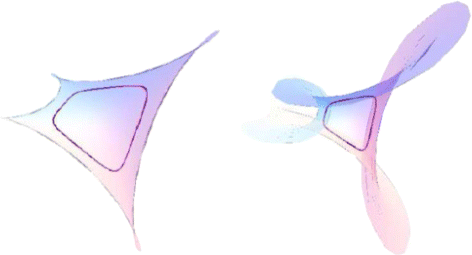Fig. 7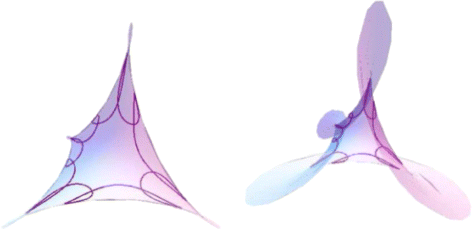Fig. 8

Typically, even at their singularities, maximal surfaces can be described as smooth graphs of functions over domains in the horizontal spacelike coordinate plane of $$\mathbb {R}^{2,1}$$ (see [4,7,8] for example), and thus Theorem 3.1 will apply to maximal surfaces.

### 2.7 Example: rotated cycloids

In the case of surfaces in $$\mathbb {R}^{3}$$, magnetic geodesics will generally not avoid singular sets on those surfaces, and the final example here illustrates this. We consider rotated cycloids in $$\mathbb {R}^{3}$$, which have cuspidal edge singularities. We choose the following parametrization

$$S(u,v)=((2+\cos u) \cos v, (2+\cos u) \sin v, u-\sin u).$$

The system becomes

\begin{aligned} c=&2u^{\prime2}(1-\cos u)+v^{\prime2}(2+\cos u)^{2}, \\ c \kappa=& \left(u^{\prime\prime}v'-v^{\prime\prime}u'\right)\sqrt{2}\sqrt{1-\cos u}(2+\cos u)\\ &+v^{\prime3}\frac{\sin u(2+\cos u)^{2}}{\sqrt{2}\sqrt{1-\cos u}} \\ &+u^{\prime2}v'\frac{(6-3\cos u)\sin u}{\sqrt{2}\sqrt{1-\cos u}}. \end{aligned}

An example of a magnetic geodesic that meets the singular set is shown in Fig. 9.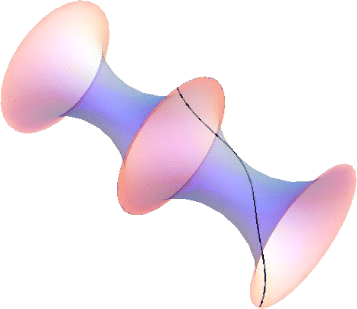Fig. 9

## Restrictions for tangency directions of magnetic geodesics passing through a singularity

In our numerical investigations of magnetic geodesics on the maximal Enneper surface we have seen that magnetic geodesics avoid the singular set of the surface. In this section we will prove a result that helps explain this behavior not only on arbitrary maximal surfaces, but on general spacelike surfaces in $$\mathbb {R}^{2,1}$$ at points where the tangent planes degenerate to become lightlike. More precisely, we will consider the case that the tangent plane T p M becomes lightlike and the surface is a graph of a function over a domain $$\mathcal {U}$$ with immersable boundary $$\partial \mathcal {U}$$ in the horizontal spacelike coordinate plane of $$\mathbb {R}^{2,1}$$ whose second derivatives are finite and not all zero at the projection of p into $$\overline {\mathcal {U}}$$.

This is the content of the following theorem:

### Theorem 3.1

Suppose that (M,g) is an almost-everywhere-spacelike smooth surface in $$\mathbb {R}^{2,1}$$ that becomes singular at a non-flat point pM.

Then there are only at most six directions within T p M to which any magnetic geodesic meeting p with C 1 regularity and bounded geodesic curvature must be tangent. Two of these at most six directions are the lightlike directions.

### Proof

We may parametrize the surface as a graph, that is S(u,v)=(u,v,f(u,v)) for some function f(u,v), and we can consider a curve γ(t)=S(u(t),v(t)). The surface is spacelike, with the exception of a measure zero set in the surface at which the tangent planes are lightlike. Without loss of generality, we assume

1. 1.

the tangent plane at u=v=0 is lightlike,

2. 2.

the surface is placed in $$\mathbb {R}^{2,1}$$ in such a way that

$$f(0,0)=0,\qquad f_{u}(0,0)=1,\qquad f_{v}(0,0)=0,$$
3. 3.

the curve γ(t) on the surface satisfies

$$\gamma(0)=S(0,0),\qquad u'(0)=\cos\theta,\qquad v'(0)=\sin\theta$$

for some value of $$\theta \in \mathbb {R} \setminus \pi \mathbb {Z}$$,

4. 4.

the tangent planes to f at the points γ(t) for t>0 are spacelike.

We assume that γ is a magnetic geodesic, thus 〈γ ,γ 〉 is a positive constant for t>0. We set

$$h=\left(1-f_{u}^{2}-f_{v}^{2}\right)^{-1} \;, \;\;\; R=f_{uu}u^{\prime2}+2f_{uv}u'v'+f_{vv}v^{\prime2} \;.$$

First, we examine the limiting behavior of u ′′(t) and v ′′(t) as t approaches 0. Because 〈γ ,γ 〉 is constant for t>0, by property (3) above we have 〈γ ,γ 〉= sin2θ for all t≥0. We can assume |n|=1 for t>0. We then have

$$\left\langle \gamma^{\prime\prime}, \gamma' \right\rangle = 0$$
(3.1)

and, by Proposition 2.5,

$$\left\langle \gamma^{\prime\prime}, \gamma' \times n \right\rangle = \kappa \sin^{2} \theta \;.$$
(3.2)

Writing

$$\gamma(t) = (u(t),v(t),f(u(t),v(t)))$$

and using

$${}\gamma' = \left(u',v',f_{u} u'+f_{v} v'\right) \;, \;\;\; \gamma^{\prime\prime} = \left(u^{\prime\prime},v^{\prime\prime},R+f_{u} u^{\prime\prime}+f_{v} v^{\prime\prime}\right) \;,$$

we can take the limit as t→0 in Eq. 3.1 to obtain the finite limit

\begin{aligned} {\lim}_{t \to 0} \left(A u^{\prime\prime} + B v^{\prime\prime}\right)=\cos \theta \cdot R|_{t=0} \;, \;\;\;\; A = \left(1-f_{u}^{2}\right) u'\\ - f_{u}f_{v} v' \;, B= \left(1-f_{v}^{2}\right) v' - f_{u}f_{v} u' \;. \end{aligned}

Noting that A| t=0=0 and B| t=0= sinθ≠0, we see that only these two cases can occur:

1. 1.

u ′′ is bounded at t=0 and $${\lim }_{t \to 0} v^{\prime \prime } = \cot \theta \cdot R|_{t=0}$$, or

2. 2.

there exists a sequence t j >0 converging to zero so that |u ′′(t j )| diverges to infinity and |v ′′(t j )/u ′′(t j )| converges to zero as j.

In the second case, we can obtain the conclusion by examining

$$u^{\prime\prime}(t_{j}) \left(A|_{t=t_{j}} + (B|_{t=t_{j}})\frac{v^{\prime\prime}(t_{j})}{u^{\prime\prime}(t_{j})} \right)$$

as j.

Note that

$$n = \sqrt{h} \left(f_{u},f_{v},1\right) \;,$$

so

\begin{aligned} \gamma' \times n &= \sqrt{h} \left(f_{v} \left(f_{u} u'+f_{v} v'\right) -v',u'-f_{u} \left(f_{u} u'+f_{v} v'\right),\right.\\ &\left.\quad u'f_{v}-v'f_{u}\right) \;. \end{aligned}

Examining the behavior as t→0 of Eq. 3.2, we see that

$${\mathcal T}:= \sqrt{h^{-1}} (u' v^{\prime\prime}-v' u^{\prime\prime}) + \sqrt{h} R \left(v' f_{u}-u' f_{v}\right)$$

is bounded near t=0.

In the first case (1) above with bounded u ′′, $${\mathcal T}$$ converges asymptotically to $$\sqrt {h} R \sin \theta$$, and this can be bounded only if R| t=0=0.

In the second case (2) above with unbounded u ′′, we can write $${\mathcal T}$$ at t j as

$${} \left(\!u^{\prime\prime} \sqrt{h} (-h^{-1} (v'-u' (v^{\prime\prime}/u^{\prime\prime})) +(R/u^{\prime\prime}) (v' f_{u}-u' f_{v}))\right)|_{t=t_{j}} \;.$$

Since u (t j ) and v (t j ) are bounded, and v ′′(t j )/u ′′(t j ) and h −1(t j ) converge to zero, and since $$(v' f_{u}-u' f_{v})|_{t=t_{j}}\phantom {\dot {i}\!}$$ converges to sinθ, as j, this term is asymptotically equal to $$(\sqrt {h} R \sin \theta)|_{t=t_{j}}$$ were R| t=0≠0, and again we conclude $${\mathcal T}$$ is bounded only if R| t=0=0.

Thus, in either case, we must have

$$\left(f_{uu}\cos^{2}\theta+2f_{uv}\cos\theta\sin\theta+f_{vv}\sin^{2}\theta\right)\big|_{u=v=0} = 0 \;.$$
(3.3)

If f vv ≠0, resp. f uu ≠0, the angle θ must satisfy

\begin{aligned} &\tan\theta=\frac{-f_{uv}\pm\sqrt{f_{uv}^{2}-f_{uu}f_{vv}}}{f_{vv}}\bigg|_{u=v=0} \;, \;\;\; \text{resp.} \;\;\;\\ &\cot\theta=\frac{-f_{uv}\pm\sqrt{f_{uv}^{2}-f_{uu}f_{vv}}}{f_{uu}}\bigg|_{u=v=0} \;. \end{aligned}
(3.4)

If f uu =f vv =0, then θ=π/2+k π for some integer k.

Thus there are at most four possible values for the angle θ [ 0,2π) in addition to θ=0,π for which the magnetic geodesic can approach the singular point p. □

### Remarks 3.2

Theorem 3.1 can be generalized to almost-everywhere-spacelike submanifolds of general dimensional Minkowski spaces, with the corresponding conclusion being that generically the possible directions in which a magnetic geodesic can approach a point with a lightlike tangent space form a subset in the space of all directions that has codimension at least 1.

## References

1. 1

Branding, V, Hanisch, F: Magnetic geodesics via the heat flow. arxiv:1411.6848, to appear In: Asian Journal of Mathematics. https://arxiv.org/abs/1411.6848.

2. 2

Contreras, G, Macarini, L, Paternain, G: Periodic orbits for exact magnetic flows on surfaces. Int. Math. Res. Not. 8, 361–387 (2004).

3. 3

Fujimori, S, Saji, K, Umehara, M, Yamada, K: Singularities of maximal surfaces. Math. Zeit. 259, 827–848 (2008).

4. 4

Fujimori, S, Kim, YW, Koh, S-E, Rossman, W, Shin, H, Umehara, M, Yamada, K, Yang, S-D: Zero mean curvature surfaces in Lorentz-Minkowski 3-space and 2-dimensional fluid dynamics. Math. J. Okayama Univ. 57, 173–200 (2015).

5. 5

Ginzburg, V: On closed trajectories of a charge in a magnetic field. Appl. Symplectic Geom. Contact Symplectic Geom. (Camb). 8, 131–148 (1994).

6. 6

Ginzburg, VL: On the existence and non-existence of closed trajectories for some Hamiltonian flows. Mathematische Zeitschrift. 223(3), 397–409 (1996).

7. 7

Gu, CH: The extremal surfces in the 3-dimensional Minkowski spaces. Acta Math. Sinica (new series). 1(2), 173–180 (1985).

8. 8

Klyachin, VA: Zero mean curvature surfaces of mixed type in Minkowski space. Izv. Math. 67, 209–224 (2003).

9. 9

Kobayashi, O: Maximal Surfaces in the 3-Dimensional Minkowski Space L3. Tokyo J. Math. 6(2), 297–309 (1983).

10. 10

Miranda, JAG: Generic properties for magnetic flows on surfaces. Nonlinearity. 19(8), 1849–1874 (2006).

11. 11

Schneider, M: Closed magnetic geodesics on S2. J. Differential Geom. 87, 343–388 (2011).

12. 12

Schneider, M: Closed magnetic geodesics on closed hyperbolic Riemann surfaces. Proc. Lond. Math. Soc. 105(3), 424–446 (2012).

13. 13

Schneider, M, Rosenberg, H: Embedded constant curvature curves on convex surfaces. Pac. J. Math. 253, 213–219 (2011).

14. 14

Taimanov, IA: Periodic magnetic geodesics on almost every energy level via variational methods. Regul. Chaotic Dyn. 15(4–5), 598–605 (2010).

Download references

### Funding

This research was supported by the joint Austrian-Japanese grant I1671-N26: Transformations and Singularities. The first author was also supported by the Austrian Science Fund (FWF) through the START-Project Y963-N35 of Michael Eichmair.

### Competing interests

Both authors declare that they have no competing interests.

### Authors’ contributions

Both authors read and approved the final manuscript.

## Author information

Authors

### Corresponding author

Correspondence to Volker Branding.

## Rights and permissions

Open Access This article is distributed under the terms of the Creative Commons Attribution 4.0 International License (http://creativecommons.org/licenses/by/4.0/), which permits unrestricted use, distribution, and reproduction in any medium, provided you give appropriate credit to the original author(s) and the source, provide a link to the Creative Commons license, and indicate if changes were made.

Reprints and Permissions